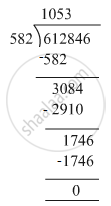# Divide and check your answer by the corresponding multiplication in the following: 612846 ​÷ 582 - Mathematics

Fill in the Blanks

Divide and check your answer by the corresponding multiplication in the following:

612846 ​÷ 582

#### SolutionDividend = 612846 , Divisor = 582, Quotient = 1053 , Remainder = 0
Check : Divisor × Quotient + Remainder= 582 × 1053 + 0
= 612846
=Dividend
Hence, Dividend = Divisor × Quotient + Remainderotient + Remainder

Is there an error in this question or solution?

#### APPEARS IN

RS Aggarwal Class 6 Mathematics
Chapter 3 Whole Numbers
Exercise 3E | Q 1.4 | Page 56